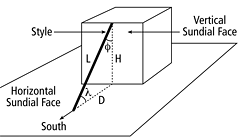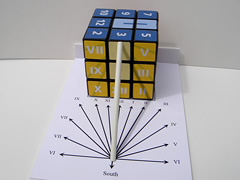In common sundial designs, the sun casts a shadow from its style onto a flat surface marked with lines indicating the hours of the day. As the sun moves across the sky, the shadow-edge progressively aligns with different hour-lines on the plate. Such designs rely on the style being aligned with the axis of the Earth's rotation.

The style (a thin rod or a sharp, straight edge) should be parallel to the Earth's axis of rotation, and point to the celestial pole. The surface where the shadow is cast is called the 'dial face' or 'dial plate' (often shortened to 'face').

Vertical Sundials

In the common 'Vertical Sundial', the shadow-receiving plane is aligned vertically. The style's angle with vertical 'φ' must equal the co-latitude, which is 90° minus the sundial's geographical latitude 'λ':

φ = 90° – λ

However, the line of shadow does not move uniformly on the face: the sundial is not equiangular. If the face of the vertical dial points directly South, the angle of the hour-lines is instead described by the formula:

tan(θ) = cos(λ) × tan(15° × t)

where 'λ' is the sundial's geographical latitude, 'θ' is the angle between a given hour-line and the noon hour-line (which always points due North) on the plane, and 't' is the number of hours before or after noon. For example, the angle θ of the 3 pm hour-line would equal the arctangent of cos(λ), since tan(45°) = 1. Interestingly, the shadow moves counter-clockwise on a South-facing vertical dial, whereas it runs clockwise on horizontal and equatorial dials.

Horizontal Sundials

In the common 'Horizontal Sundial', the shadow-receiving plane is aligned horizontally. The style's angle with the plane must equal the sundial's geographical latitude 'λ'.

As is also the case for the vertical sundial, the line of shadow does not move uniformly on the plane: the sundial is not equiangular. If the style points directly North, the angle of the hour-lines is instead described by the formula:

tan(θ) = sin(λ) × tan(15° × t)

where 'λ' is the sundial's geographical latitude, 'θ' is the angle between a given hour-line and the noon hour-line (which always points due North) on the plane, and 't' is the number of hours before or after noon. For example, the angle θ of the 3 pm hour-line would equal the arctangent of sin(λ), since tan(45°) = 1. Interestingly, the shadow moves clockwise on horizontal and equatorial dials, whereas it runs counter-clockwise on a South-facing vertical dial.

Note that the angle θ between a given hour-line and the noon hour-line is not the same for the vertical and horizontal sundials.

Combined Sundials

A vertical and a horizontal sundial can be combined by sharing the same style, because their style angles are complementary i.e. they sum up to 90°. The horizontal sundial plane can also be used as a base plate for the cube. In that case, the common style should only be fastened onto the plane and not onto the cube. The common style length 'L' and the distance of the style far end from the cube 'D' are given by:

L = H / sin(λ) and D = H / tan(λ)

where 'λ' is the sundial's geographical latitude and 'H' is the cube height, usually equal to 57 mm for a regular Rubik's cube.

The style can be made from a 3 mm diameter plastic rod, which should be bevelled at both ends. The rod lower end can then be glued onto the base plate whereas the upper end simply leans against the top center cubie.There are three adjustments to calculate local clock time from a sundial reading:

1. Summer (daylight saving) time correction

2. Time zone (Longitude) correction

3. Equation of time correction

### Sundial Cube

The Sundial Cube is used to measure the time by the position of the sun. This is a 'Vertical Sundial' with time displayed on the cube front face. However, to be fully functional as a real sundial, the cube needs an accessory, which is a 'shadow maker', called a gnomon or style . When the cube is not used as a sundial, it can nevertheless be used as a Super Cube, which is a design variation of a Rubik's Cube with marker strokes drawn on some stickers to show the orientation of side parts. The sundial cube noon lines can then be used as Super Cube strokes.

The vertical sundial cube can be read in six capital cities, all around the globe: London (Great Britain), Roma (Italy), New Delhi (India), Beijing (People's Republic of China), Seoul (South Korea) and Riyadh (Saudi Arabia). Six kinds of numerals are used on the cube: Arabic, Roman, Hindi (Devanagari), Chinese/Japanese, Korean (Hangul) and Arabic-Indic numerals. Moreover, the computed angle between a given hour-line and the noon hour-line is different for each capital city, making this cube a precise solar clock.

London, Great Britain (blue face)

Arabic numerals (1-12):

1, 2, 3, 4, 5, 6, 7, 8, 9, 10, 11, 12.

Latitude (λ), longitude:

51° 30′ N, 0° 08′ W.

Style angles:

φ = 38.5°, λ = 51.5°.

Style measures:

H = 57 mm, D = 45 mm, L = 73 mm.

Vertical sundial angles:

θ (t=1h): 9.5°.

θ (t=2h): 19.8°.

θ (t=3h): 31.9°.

θ (t=4h): 47.2°.

θ (t=5h): 66.7°.

Horizontal sundial angles:

θ (t=1h): 11.8°.

θ (t=2h): 24.3°.

θ (t=3h): 38.1°.

θ (t=4h): 53.6°.

θ (t=5h): 71.1°.

Roma, Italy (yellow face)

Roman numerals (1-12):

I, II, III, IV, V, VI, VII, VIII, IX, X, XI, XII.

Latitude (λ), longitude:

41° 54′ N, 12° 29′ E.

Style angles:

φ = 48.0°, λ = 42.0°.

Style measures:

H = 57 mm, D = 64 mm, L = 85 mm.

Vertical sundial angles:

θ (t=1h): 11.3°.

θ (t=2h): 23.3°.

θ (t=3h): 36.7°.

θ (t=4h): 52.2°.

θ (t=5h): 70.2°.

Horizontal sundial angles:

θ (t=1h): 10.2°.

θ (t=2h): 21.1°.

θ (t=3h): 33.8°.

θ (t=4h): 49.2°.

θ (t=5h): 68.2°.

New Dehli, India (orange face)

Hindi (Devanagari) numerals (1-12):

१, २, ३, ४, ५, ६, ७, ८, ९, १०, ११, १२.

Latitude (λ), longitude:

28° 38′ N, 77° 13′ E.

Style angles:

φ = 61.3°, λ = 28.7°.

Style measures:

H = 57 mm, D = 104 mm, L = 119 mm.

Vertical sundial angles:

θ (t=1h): 13.2°.

θ (t=2h): 26.9°.

θ (t=3h): 41.3°.

θ (t=4h): 56.7°.

θ (t=5h): 73.0°.

Horizontal sundial angles:

θ (t=1h): 7.3°.

θ (t=2h): 15.5°.

θ (t=3h): 25.7°.

θ (t=4h): 39.8°.

θ (t=5h): 60.8°.

Beijing, People's Republic of China (red face)

Chinese/Japanese numerals (1-12):

Latitude (λ), longitude:

39° 54′ N, 116° 23′ E.

Style angles:

φ = 50.0°, λ = 40.0°.

Style measures:

H = 57 mm, D = 68 mm, L = 89 mm.

Vertical sundial angles:

θ (t=1h): 11.6°.

θ (t=2h): 23.9°.

θ (t=3h): 37.5°.

θ (t=4h): 53.0°.

θ (t=5h): 70.7°.

Horizontal sundial angles:

θ (t=1h): 9.8°.

θ (t=2h): 20.4°.

θ (t=3h): 32.8°.

θ (t=4h): 48.1°.

θ (t=5h): 67.4°.

Seoul, South Korea (pink face)

Korean (Hangul) numerals (1-12):

하나, 둘, 셋, 넷, 다섯, 여섯, 일곱, 여덟, 아홉, 열, 열하나, 열둘.

Latitude (λ), longitude:

37° 33′ N, 126° 59′ E.

Style angles:

φ = 52.5°, λ = 37.5°.

Style measures:

H = 57 mm, D = 74 mm, L = 94 mm.

Vertical sundial angles:

θ (t=1h): 12.0°.

θ (t=2h): 24.6°.

θ (t=3h): 38.4°.

θ (t=4h): 53.9°.

θ (t=5h): 71.3°.

Horizontal sundial angles:

θ (t=1h): 9.3°.

θ (t=2h): 19.4°.

θ (t=3h): 31.3°.

θ (t=4h): 46.5°.

θ (t=5h): 66.2°.

Riyadh, Saudi Arabia (green face)

Arabic-Indic numerals (1-12):

١, ٢, ٣, ٤, ٥, ٦, ٧, ٨, ٩, ١٠, ١١, ١٢.

Latitude (λ), longitude:

24° 43′ N, 46° 43′ E.

Style angles:

φ = 65.3°, λ = 24.7°.

Style measures:

H = 57 mm, D = 124 mm, L = 136 mm.

Vertical sundial angles:

θ (t=1h): 13.7°.

θ (t=2h): 27.7°.

θ (t=3h): 42.3°.

θ (t=4h): 57.6°.

θ (t=5h): 73.6°.

Horizontal sundial angles:

θ (t=1h): 6.4°.

θ (t=2h): 13.6°.

θ (t=3h): 22.7°.

θ (t=4h): 35.9°.

θ (t=5h): 57.3°.

The layout of the Sundial Cube was created in 2008 by André Boulouard and Walter Randelshofer.

Faces of the cube: 1024 x 1024, 2048 x 2048

Enlarged view